.

# 一 特徵工程介紹（Feature Engineering）

## 1 定義及意義

### （1）定義

• 特徵工程（Feature Engineering）特徵工程是將原始資料轉化成更好的表達問題本質的特徵的過程，使得將這些特徵運用到預測模型中能提高對不可見資料的模型預測精度。
• 特徵工程簡單講就是發現對因變數y有明顯影響作用的特徵，通常稱自變數x為特徵，特徵工程的目的是發現重要特徵。
• 如何能夠分解和聚合原始資料，以更好的表達問題的本質？這是做特徵工程的目的。

“feature engineering is manually designing what the input x’s should be.”
“you have to turn your inputs into things the algorithm can understand.”

• 特徵工程是資料探勘模型開發中最耗時、最重要的一步。

### （2）意義

• 特徵工程是一個包含內容很多的主題，也被認為是成功應用機器學習的一個很重要的環節。如何充分利用資料進行預測建模就是特徵工程要解決的問題！

“實際上，所有機器學習演算法的成功取決於如何呈現資料。”
“特徵工程是一個看起來不值得在任何論文或者書籍中被探討的一個主題。但是他卻對機器學習的成功與否起著至關重要的作用。機器學習演算法很多都是由於建立一個學習器能夠理解的工程化特徵而獲得成功的。”——ScottLocklin，in “Neglected machine learning ideas”

• 資料中的特徵對預測的模型和獲得的結果有著直接的影響。可以這樣認為，特徵選擇和準備越好，獲得的結果也就越好。這是正確的，但也存在誤導。預測的結果其實取決於許多相關的屬性：比如說能獲得的資料、準備好的特徵以及模型的選擇。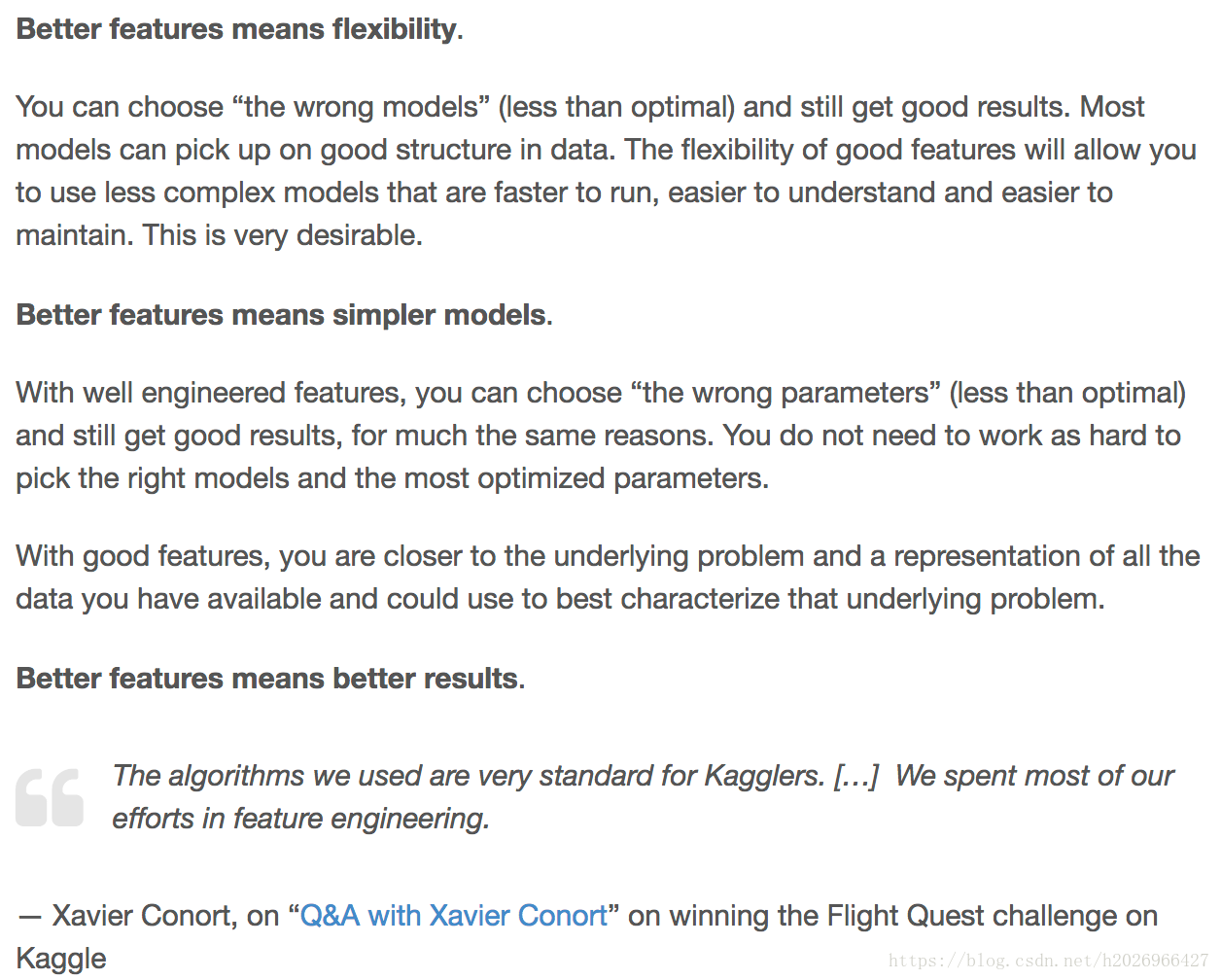### （3）相關概念

Feature Selection：From many features to a few that are useful
Feature Extraction：The automatic construction of new features from raw data.
Feature Construction：The manual construction of new features from raw data.
Feature Importance：An estimate of the usefulness of a feature.

## 2 流程及方法

• 1.圖一是基本的資料探勘場景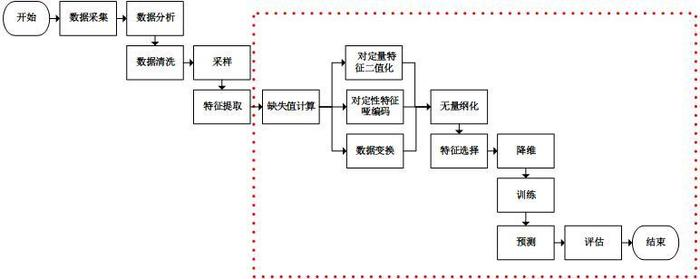• 2.圖二是特徵工程的迭代過程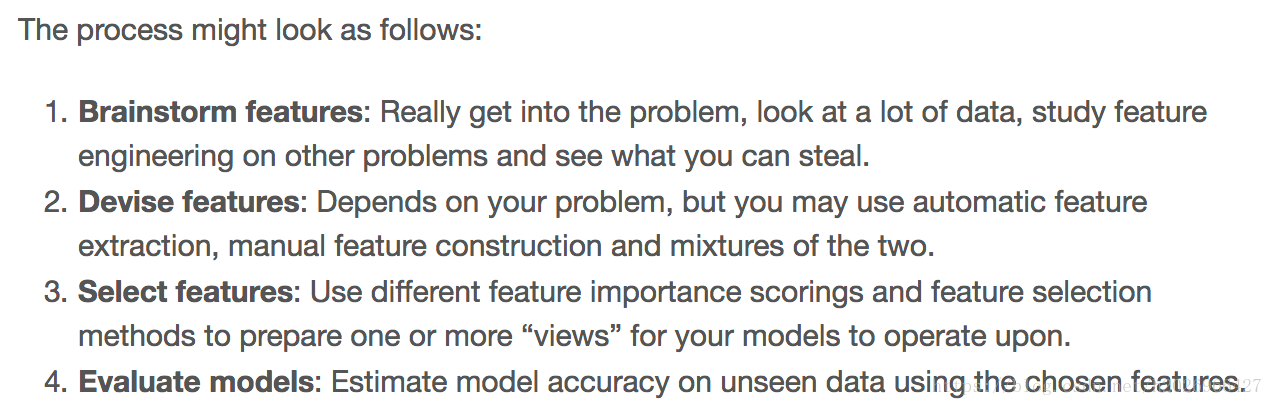• 3.圖三是特徵工程的常見方法和步驟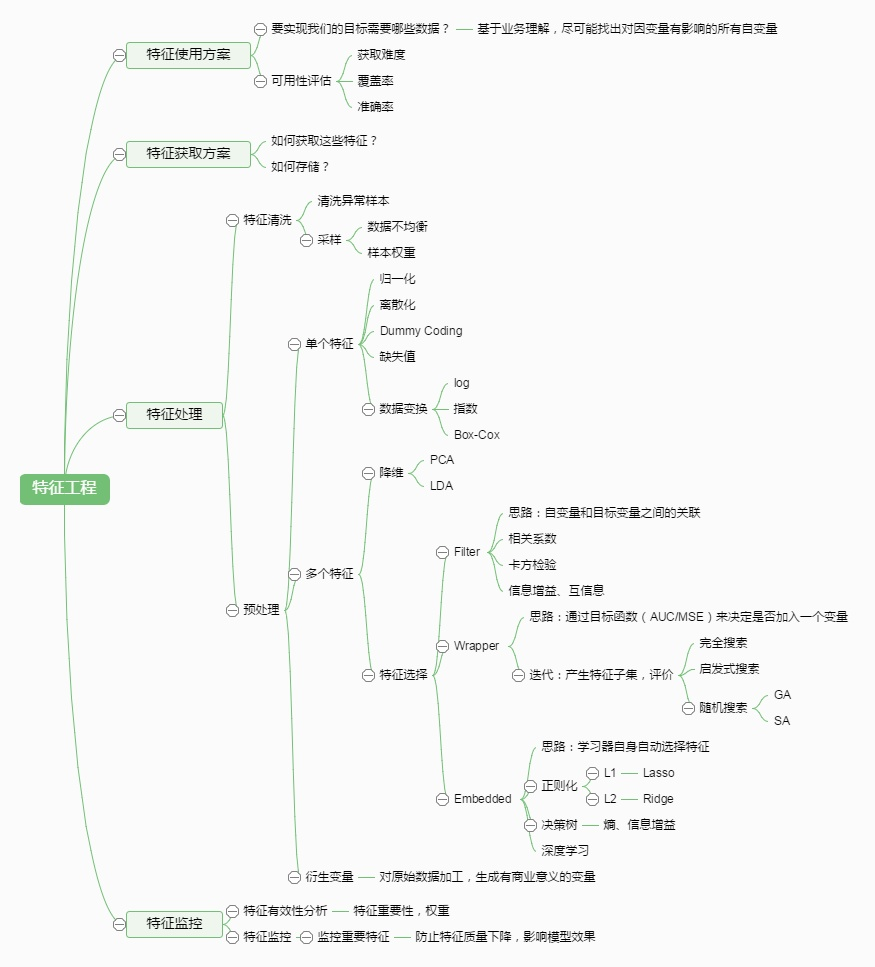# 二 資料獲取

①資料獲取途徑

• 如何獲取特徵（介面呼叫or自己清洗or/github資源下載等）
• 如何儲存？（/data/csv/txt/array/Dataframe//其他常用分散式）

②資料可用性評估

• 獲取難度
• 覆蓋率
• 準確率

③特徵維度

# 三 資料描述（Feature Describe）

• 存在缺失值：缺失值需要補充。
• 不屬於同一量綱：即特徵的規格不一樣，不能夠放在一起比較。
• 資訊冗餘：對於某些定量特徵，其包含的有效資訊為區間劃分，例如學習成績，假若只關心“及格”或不“及格”，那麼需要將定量的考分，轉換成“1”和“0”表示及格和未及格。
• 定性特徵不能直接使用：某些機器學習演算法和模型只能接受定量特徵的輸入，那麼需要將定性特徵轉換為定量特徵。
• 資訊利用率低：不同的機器學習演算法和模型對資料中資訊的利用是不同的。

# 四 特徵處理（Feature Processing）

①資料預處理。即資料的清洗工作，主要為缺失值、異常值、錯誤值、資料格式、取樣度等問題的處理。
②特徵轉換。即連續變數、離散變數、時間序列等的轉換，便於入模。

## 1 資料預處理

【特徵工程】python特徵預處理大全

### （1）缺失值處理

#### 2）缺失值填充（fillna）

##### ①用固定值填充

``````data['灰度分'] = data['灰度分'].fillna('-99')
``````
##### ②用均值填充

``````data['灰度分'] = data['灰度分'].fillna(data['灰度分'].mean()))
``````
##### ③用眾數填充

``````data['灰度分'] = data['灰度分'].fillna(data['灰度分'].mode()))
``````
##### ④用上下資料進行填充
• 用前一個數據進行填充
``````data['灰度分'] = data['灰度分'].fillna(method='pad')
``````
• 用後一個數據進行填充
``````data['灰度分'] = data['灰度分'].fillna(method='bfill')
``````
##### ⑤用插值法填充
``````data['灰度分'] = data['灰度分'].interpolate()
``````
##### ⑥用KNN進行填充
``````from fancyimpute import BiScaler, KNN, NuclearNormMinimization, SoftImpute
dataset = KNN(k=3).complete(dataset)
``````
##### ⑦random forest進行填充
``````from sklearn.ensemble import RandomForestRegressor
zero_columns_2 = ['機構查詢數量', '直接聯絡人數量', '直接聯絡人在黑名單數量', '間接聯絡人在黑名單數量',
'引起黑名單的直接聯絡人數量', '引起黑名單的直接聯絡人佔比']
#將出現空值的除了預測的列全部取出來，不用於訓練
dataset_list2 = [x for x in dataset if x not in zero_columns_2]
dataset_2 = dataset[dataset_list2]
# 取出灰度分不為空的全部樣本進行訓練
know = dataset_2[dataset_2['灰度分'].notnull()]
print(know.shape) #26417, 54
# 取出灰度分為空的樣本用於填充空值
unknow = dataset_2[dataset_2['灰度分'].isnull()]
print(unknow.shape) #2078, 54
y = ['灰度分']
x = 
know_x2 = know.copy()
know_y2 = know.copy()
print(know_y2.shape)
#
know_x2.drop(know_x2.columns[x], axis=1, inplace=True)
print(know_y2.shape)
print(know_x2.shape)
#
know_y2 = know[y]
# RandomForestRegressor
rfr = RandomForestRegressor(random_state=666, n_estimators=2000, n_jobs=-1)
rfr.fit(know_x2, know_y2)
# 填充為空的樣本
unknow_x2 = unknow.copy()
unknow_x2.drop(unknow_x2.columns[x], axis=1, inplace=True)
print(unknow_x2.shape) #(2078, 53)
unknow_y2 = rfr.predict(unknow_x2)
unknow_y2 = pd.DataFrame(unknow_y2, columns=['灰度分'])
``````
##### ⑧使用fancyimpute包中的其他方法
``````# matrix completion using convex optimization to find low-rank solution
# that still matches observed values. Slow!
X_filled_nnm = NuclearNormMinimization().complete(X_incomplete)
``````
``````# Instead of solving the nuclear norm objective directly, instead
# induce sparsity using singular value thresholding
X_filled_softimpute = SoftImpute().complete(X_incomplete_normalized)
``````
``````# print mean squared error for the three imputation methods above
# print mean squared error for the three imputation methods above
print("Nuclear norm minimization MSE: %f" % nnm_mse)
print("SoftImpute MSE: %f" % softImpute_mse)
print("knnImpute MSE: %f" % knn_mse)
``````
##### ⑨缺失值作為資料的一部分不填充

LightGBM和XGBoost都能將NaN作為資料的一部分進行學習，所以不需要處理缺失值。

### （2）異常值處理

#### 1）特徵異常平滑

• 基於統計的異常點檢測演算法
例如極差，四分位數間距，均差，標準差等，這種方法適合於挖掘單變數的數值型資料。
• 基於距離的異常點檢測演算法
主要通過距離方法來檢測異常點，將資料集中與大多數點之間距離大於某個閾值的點視為異常點，主要使用的距離度量方法有絕對距離(曼哈頓距離)、歐氏距離和馬氏距離等方法。
• 基於密度的異常點檢測演算法
考察當前點周圍密度，可以發現區域性異常點。

• 數字型別的轉換
• 數字單位的調整
• 時間格式的處理

## 2 特徵轉換

• 連續型
• 無序類別（離散）型
• 有序類別（離散）型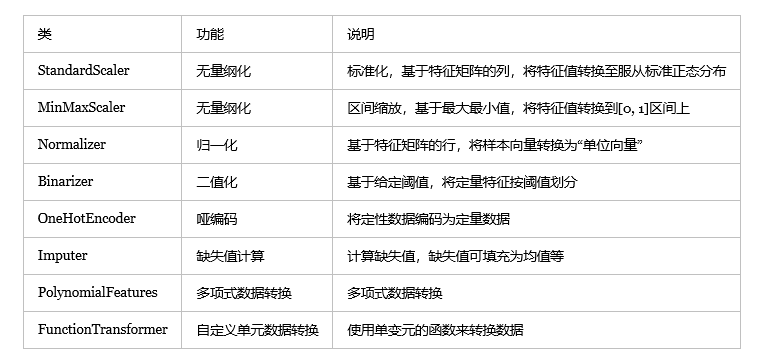### （1）連續型特徵處理

#### 3）無量綱化

##### ①標準化
###### · StandardScaler標準化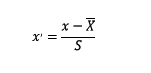``````from sklearn.preprocessing import StandardScaler
#標準化，返回值為標準化後的資料
StandardScaler().fit_transform(iris.data)
``````
##### ②歸一化
###### · 反餘切轉換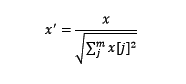``````from sklearn.preprocessing import Normalizer
#歸一化，返回值為歸一化後的資料
Normalizer().fit_transform(iris.data)
``````
##### ③區間縮放法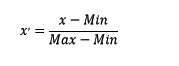``````from sklearn.preprocessing import MinMaxScaler
#區間縮放，返回值為縮放到[0, 1]區間的資料
MinMaxScaler().fit_transform(iris.data)

``````

#### 4）二值化（定量特徵）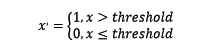``````from sklearn.preprocessing import Binarizer
#二值化，閾值設定為3，返回值為二值化後的資料
Binarizer(threshold=3).fit_transform(iris.data)
``````

#### 5）離散化分箱處理(數值型轉類別型)

• 離散特徵的增加和減少都很容易，易於模型的快速迭代；
• 稀疏向量內積乘法運算速度快，計算結果方便儲存，容易擴充套件；
• 離散化後的特徵對異常資料有很強的魯棒性模型也會更穩定；
• 離散化後可以進行特徵交叉，由M+N個變數變為M*N個變數，進一步引入非線性 提 升表達能力；
• 特徵離散化以後，起到了簡化了邏輯迴歸模型的作用，降低了模型過擬合的風險

• 等距離離散（等距分組）
顧名思義，就是離散點選取等距點。
• 等樣本點離散（等深分組）
選取的離散點保證落在每段裡的樣本點數量大致相同
• 決策樹離散化（最優分組）
決策樹離散化方法通常也是每次離散化一個連續特徵，原理如下：
單獨用此特徵和目標值y訓練一個決策樹模型，然後把訓練獲得的模型內的特徵分割點作為離散化的離散點。
• 其他離散化方法
其中，最優分組除決策樹方法以外，還可以使用卡方分箱的方法，這種方法在評分卡開發中比較常見。

### （2）離散型特徵處理

#### 1）數值化處理

• 不適用於建立預測具體數值的模型，比如線性迴歸，只能用於分類，
• 即使用於分類，也有一些模型不適合，
• 可能結果的精度不如one-hot編碼。

#### 2）啞編碼

##### ②順序性啞變數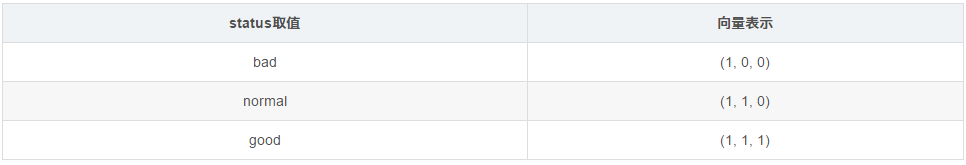（上面這種表達方式很巧妙地利用遞進表達了值之間的順序關係。）

# 五 特徵選擇（Feature Selection）

## 2 特徵選擇

### （1）定義### （2）作用

• 簡化模型，增加模型的可解釋性
• 縮短訓練時間
• 避免維度災難
• 改善模型通用性、降低過擬合

### （3）方法

• 特徵選擇演算法可以利用得分排序的方法選擇特徵，如相關性和其他特徵重要性手段；
• 更高階的方法通過試錯來搜尋特徵子集。這些方法通過建立模型，評價模型，然後自動的獲得對於目標最具預測能力的特徵子集。
• 還有一些演算法能得到特徵選擇的副產品。比如說逐步迴歸就是能夠自動的選擇特徵來構建模型。
• 正則化的方法比如lasso和嶺迴歸可以作為特徵選擇的演算法。他們在構建模型的過程中刪去或者減小不重要特徵的貢獻。（An Introduction to feature selection）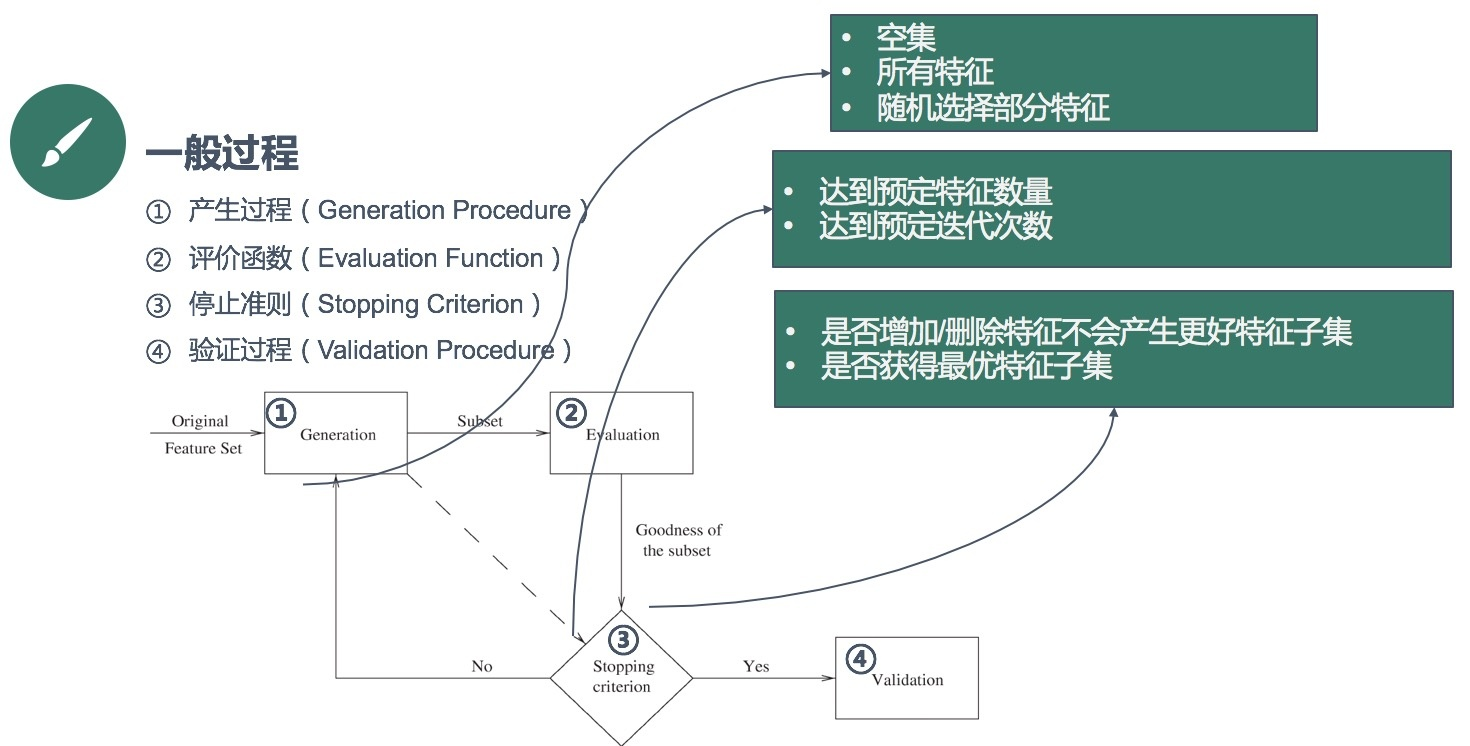• Filter：過濾法，按照發散性或者相關性對各個特徵進行評分，設定閾值或者待選擇閾值的個數，選擇特徵。
• Wrapper：包裝法，根據目標函式（通常是預測效果評分），每次選擇若干特徵，或者排除若干特徵。
• Embedded：嵌入法，先使用某些機器學習的演算法和模型進行訓練，得到各個特徵的權值係數，根據係數從大到小選擇特徵。類似於Filter方法，但是是通過訓練來確定特徵的優劣。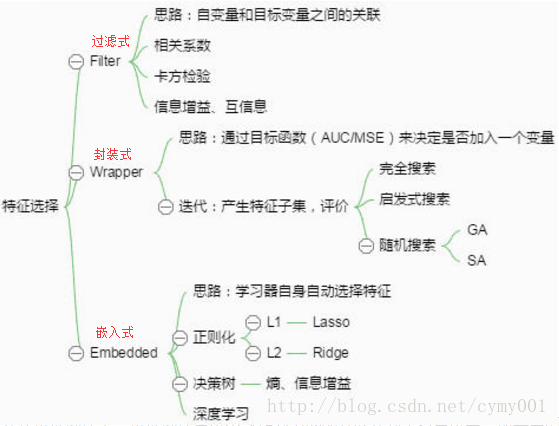#### 1）過濾式（Filter）

##### ①方差選擇法

`````` from sklearn.feature_selection import VarianceThreshold
#方差選擇法，返回值為特徵選擇後的資料
#引數threshold為方差的閾值
VarianceThreshold(threshold=3).fit_transform(iris.data)
``````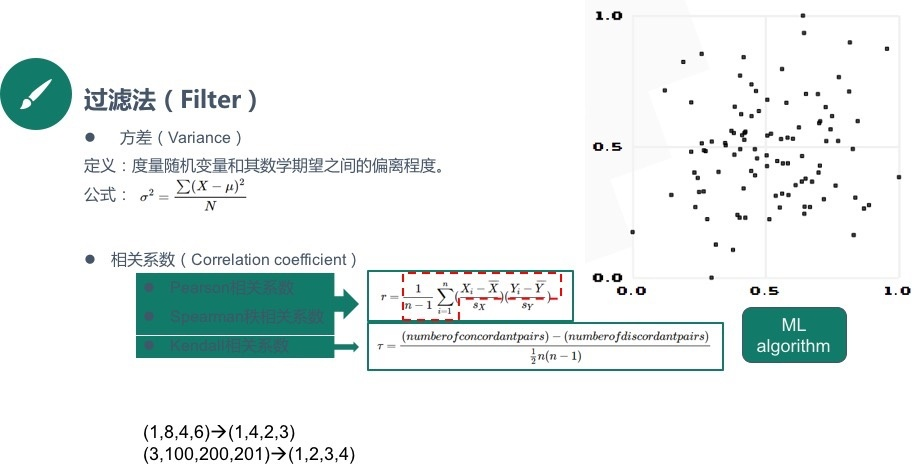##### ②相關係數法

``````from sklearn.feature_selection import SelectKBest
from scipy.stats import pearsonr
#選擇K個最好的特徵，返回選擇特徵後的資料
#第一個引數為計算評估特徵是否好的函式，該函式輸入特徵矩陣和目標向量，輸出二元組（評分，P值）的陣列，陣列第i項為第i個特徵的評分和P值。在此定義為計算相關係數
#引數k為選擇的特徵個數
SelectKBest(lambda X, Y: array(map(lambda x:pearsonr(x, Y), X.T)).T, k=2).fit_transform(iris.data, iris.target)
``````

##### ③互資訊法

Linear Discriminant Analysis(LDA，線性判別分析)：更 像一種特徵抽取方式，基本思想是將高維的特徵影到最佳鑑別向量空間，這樣就可以抽取分類資訊和達到壓縮特徵空間維數的效果。投影后的樣本在子空間有最大可分離性。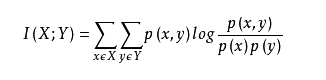``````from sklearn.feature_selection import SelectKBest
from minepy import MINE
#由於MINE的設計不是函式式的，定義mic方法將其為函式式的，返回一個二元組，二元組的第2項設定成固定的P值0.5
def mic(x, y):
m = MINE()
m.compute_score(x, y)
return (m.mic(), 0.5)
#選擇K個最好的特徵，返回特徵選擇後的資料
SelectKBest(lambda X, Y: array(map(lambda x:mic(x, Y), X.T)).T, k=2).fit_transform(iris.data, iris.target)
``````
##### ④卡方檢驗（Chi-Square）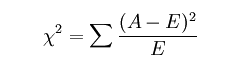``````from sklearn.feature_selection import SelectKBest
from sklearn.feature_selection import chi2
#選擇K個最好的特徵，返回選擇特徵後的資料
SelectKBest(chi2, k=2).fit_transform(iris.data, iris.target)
``````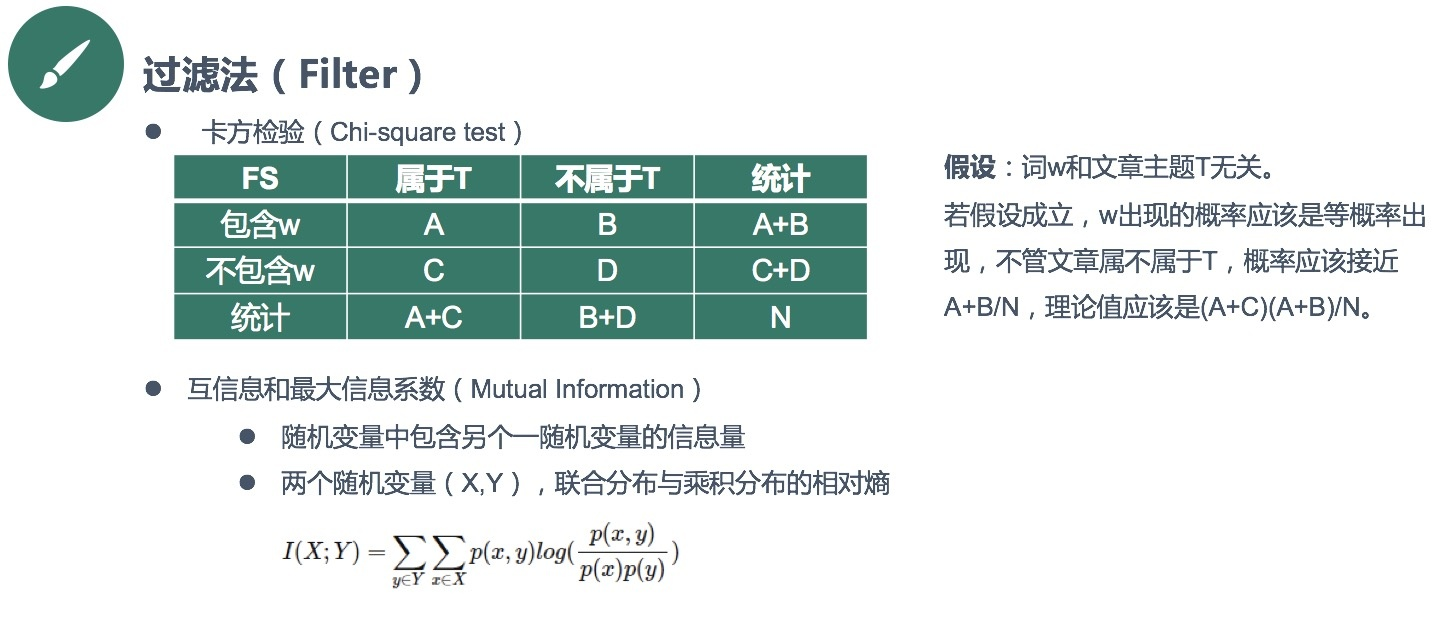#### 2）封裝式（Wrapper）

Wrapper：封裝式特徵選擇是利用學習演算法的效能評價特徵子集的優劣。因此，對於一個待評價的特徵子集，Wrapper方法需要訓練一個分類器，根據分類器的效能對該特徵子集進行評價。Wrapper方法中用以評價特徵的學習演算法是多種多樣的，例如決策樹、神經網路、貝葉斯分類器、近鄰法、支援向量機等等。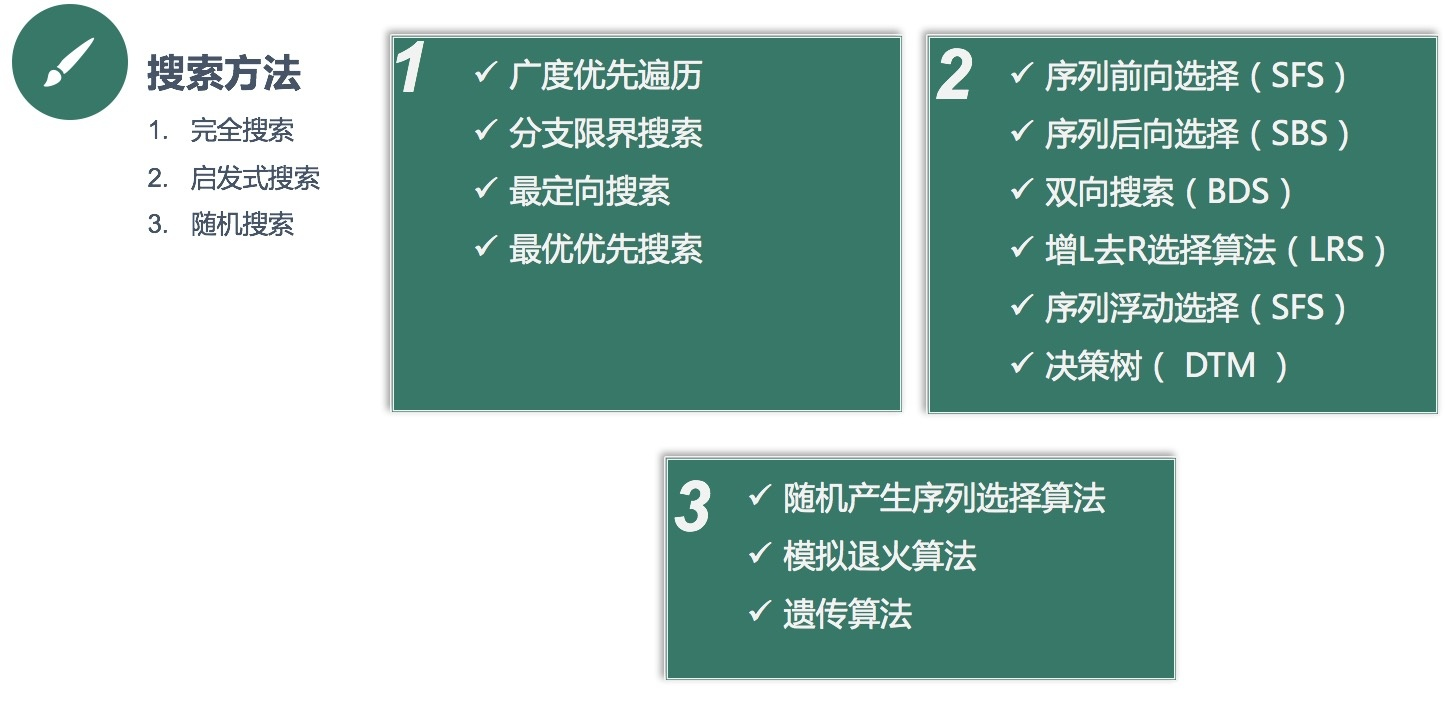##### ②啟發式搜尋[貪心]

###### · 後向剃除法

``````from sklearn.feature_selection import RFE
from sklearn.linear_model import LogisticRegression
#遞迴特徵消除法，返回特徵選擇後的資料
#引數estimator為基模型
#引數n_features_to_select為選擇的特徵個數
RFE(estimator=LogisticRegression(), n_features_to_select=2).fit_transform(iris.data, iris.target)
``````

Wrapper特點：

#### 3）嵌入式（Embedded）

• 正則化項（ L1、L2 ）
• LASSO迴歸
• 嶺迴歸（RidgeRegression）
• 決策樹
• ID3、C4.5、CART
• 深度學習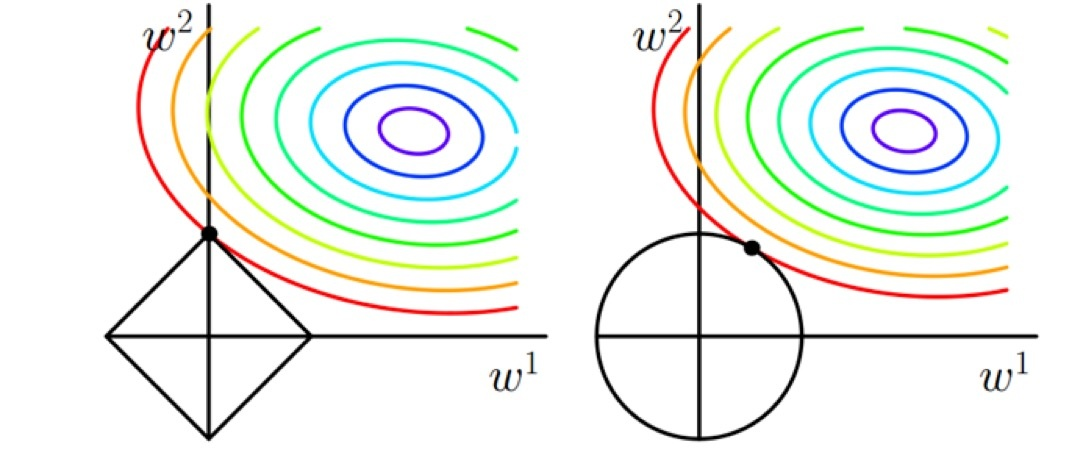##### ①基於懲罰項

``````from sklearn.feature_selection import SelectFromModel
from sklearn.linear_model import LogisticRegression
#帶L1懲罰項的邏輯迴歸作為基模型的特徵選擇
SelectFromModel(LogisticRegression(penalty="l1", C=0.1)).fit_transform(iris.data, iris.target)
``````

L1懲罰項降維的原理在於保留多個對目標值具有同等相關性的特徵中的一個，所以沒選到的特徵不代表不重要。故，可結合L2懲罰項來優化。具體操作為：若一個特徵在L1中的權值為1，選擇在L2中權值差別不大且在L1中權值為0的特徵構成同類集合，將這一集合中的特徵平分L1中的權值，故需要構建一個新的邏輯迴歸模型：

``````from sklearn.linear_model import LogisticRegression

class LR(LogisticRegression):
def __init__(self, threshold=0.01, dual=False, tol=1e-4, C=1.0,
fit_intercept=True, intercept_scaling=1, class_weight=None,
random_state=None, solver='liblinear', max_iter=100,
multi_class='ovr', verbose=0, warm_start=False, n_jobs=1):

#權值相近的閾值
self.threshold = threshold
LogisticRegression.__init__(self, penalty='l1', dual=dual, tol=tol, C=C,
fit_intercept=fit_intercept, intercept_scaling=intercept_scaling, class_weight=class_weight,
random_state=random_state, solver=solver, max_iter=max_iter,
multi_class=multi_class, verbose=verbose, warm_start=warm_start, n_jobs=n_jobs)
#使用同樣的引數建立L2邏輯迴歸
self.l2 = LogisticRegression(penalty='l2', dual=dual, tol=tol, C=C, fit_intercept=fit_intercept, intercept_scaling=intercept_scaling, class_weight = class_weight, random_state=random_state, solver=solver, max_iter=max_iter, multi_class=multi_class, verbose=verbose, warm_start=warm_start, n_jobs=n_jobs)

def fit(self, X, y, sample_weight=None):
#訓練L1邏輯迴歸
super(LR, self).fit(X, y, sample_weight=sample_weight)
self.coef_old_ = self.coef_.copy()
#訓練L2邏輯迴歸
self.l2.fit(X, y, sample_weight=sample_weight)

cntOfRow, cntOfCol = self.coef_.shape
#權值係數矩陣的行數對應目標值的種類數目
for i in range(cntOfRow):
for j in range(cntOfCol):
coef = self.coef_[i][j]
#L1邏輯迴歸的權值係數不為0
if coef != 0:
idx = [j]
#對應在L2邏輯迴歸中的權值係數
coef1 = self.l2.coef_[i][j]
for k in range(cntOfCol):
coef2 = self.l2.coef_[i][k]
#在L2邏輯迴歸中，權值係數之差小於設定的閾值，且在L1中對應的權值為0
if abs(coef1-coef2) < self.threshold and j != k and self.coef_[i][k] == 0:
idx.append(k)
#計算這一類特徵的權值係數均值
mean = coef / len(idx)
self.coef_[i][idx] = mean
return self
``````

``````from sklearn.feature_selection import SelectFromModel
#帶L1和L2懲罰項的邏輯迴歸作為基模型的特徵選擇
#引數threshold為權值係數之差的閾值
SelectFromModel(LR(threshold=0.5, C=0.1)).fit_transform(iris.data, iris.target)
``````
##### ②基於樹模型

• Lasso
• Elastic Net
• Ridge Regression
優點： 快速， 並且面向演算法。缺點： 需要調整結構和引數配置， 而這需要深入的知識和經驗。
`````` from sklearn.feature_selection import SelectFromModel
#GBDT作為基模型的特徵選擇
``````

## 3 特徵構造

### （3）方法

#### 1）簡單構造

##### ③分解類別特徵

• 二值特徵has_color： 1知道具體顏色，0表示不知道。這個可以替換item_color特徵用到更簡單的線性模型中。
• 三個二值特徵is_red、is_green和is_unknown。這個可以新增到原有特徵中用到決策樹模型中。

## 4 特徵提取

### （2）作用

​ 資料降維有以下幾點好處：
​ 1、避免維度災難，導致演算法失效，或者時間複雜度高
​ 2、避免高維資料中引入的噪聲，防止過擬合
​ 3、壓縮儲存，視覺化分析

### （3）方法

​ 不同的資料降維方法除了實現降維目標的作用，同時具有各自的特點，比如